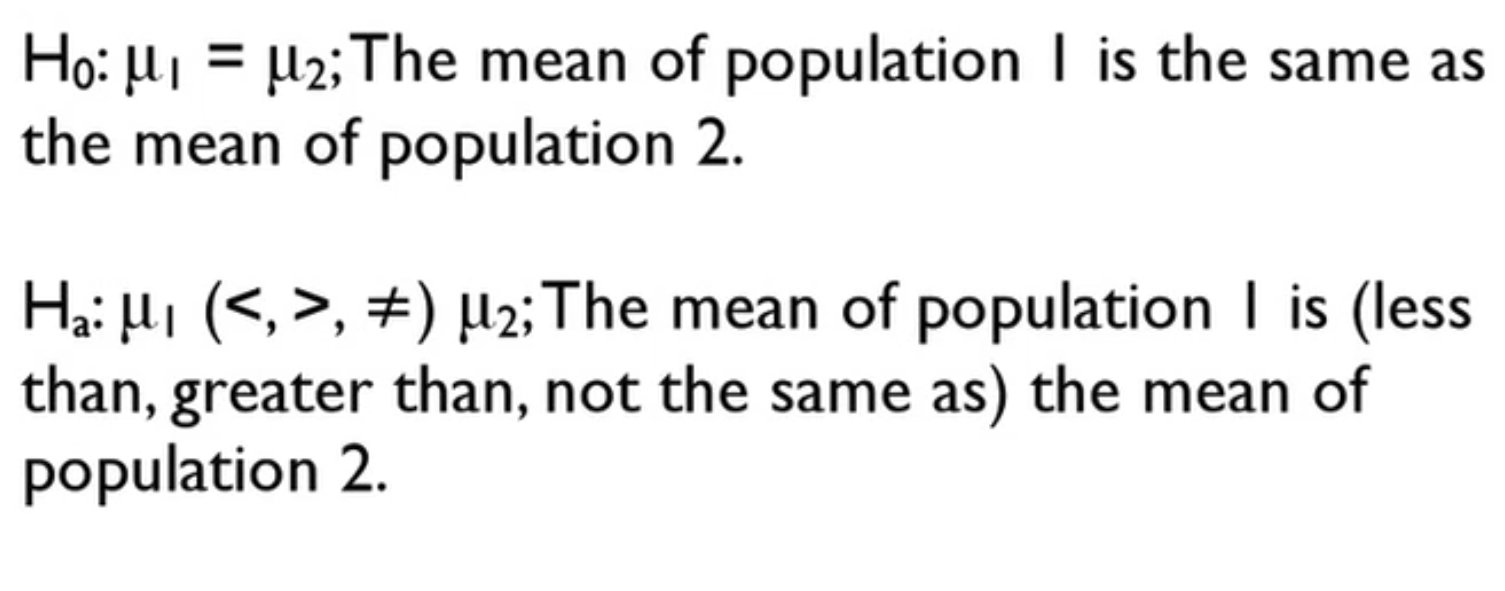# Null and Alternative Hypotheses with Two Samples

##### Rating:
(2)
• (0)
• (1)
• (0)
• (0)
• (1)
Author: Sophia Tutorial
##### Description:

This lesson will explain null and alternative hypotheses with two samples.

(more)
TutorialIn this tutorial, you're going to learn about the null and alternative hypotheses of a hypothesis test when there are two samples involved instead of just one. Specifically you will focus on:

1. Null Hypothesis With Two Samples

## 1. NULL HYPOTHESIS WITH TWO SAMPLES

In a situation where there are two samples, there are two possible scenarios that might actually be happening with your data. One is that they're independent samples, where the values from one sample don't really match or pair or affect any of the values from the other sample. The other scenario is that the samples are in fact paired together, where the first data point from the first sample actually does have a meaningful pairing with the first data point from the second sample.

That is what you call that paired data. Each pair essentially constitutes one data point instead of two different data points.

If the data are from independent samples, then the null hypothesis states that the population mean for population one and the population mean for population two are equal. The alternative hypothesis is that the means of the two populations are not the same, either that the mean of population one is smaller than that of population two, or bigger than the mean of population two, or simply not equal to the mean of population two. But notice there are two different populations and two different population means in each of these cases.• Null Hypothesis with Two Samples
• The hypothesis that there is no difference in the two population means.An example of when you would use something like this is if you were doing a controlled experiment, where you would want to compare the mean weight loss between the two populations of the treatment group and the control group. What if the data are paired together? Well, the null hypothesis in that case would simply state the true mean of the differences between those pairs is zero. So the mean of the difference between the parent data is zero.

Whereas the alternative hypothesis would say that the true mean difference is actually greater than, less than, or equal to zero.• Alternative Hypothesis with Two Samples
• The hypothesis that there is a difference in the two population meansYou might say that the true mean of the difference between husbands' heights and wives' heights. Each husband-wife pair would constitute one data point. If you had a whole list of husbands and that was paired with a whole list of wives, you would be really interested in simply the difference between the two heights, and not the actual list of the husbands and the list of wives.

Here's the thing. With the paired data, the remaining work for the matched pairs scenario, that is the conditions, the mechanics that is calculating the t-statistic and everything, and the decision and conclusion are the same as they are for a one-sample scenario. If it's matched pairs, like the husband and wife heights example, you can treat it like a one-sample scenario.

If the samples are independent and it is a two sample scenario like our weight loss example, then you have to resort to more complicated formulas for the mechanics. The conditions end up needing to be verified. The mechanics, the formulas are more complicated. And the decision and conclusion do end up following from the fact that there are two samples.

A lot of the work is different than they are in a one-sample scenario so it's important to know what the differences are between a matched pair scenario, which is apparently essentially a glorified one-sample scenario versus a two-sample scenario, which is more complicated.

The statistic and p-value are almost always calculated using technology.This tutorial demonstrated null hypotheses with two samples.  When examining an experiment or an observational study that has two groups, decide if they're independent groups, where the values from the one group aren't affected by the values from the other group, or if they're paired, where the first data point in the first list and the first data point on the second list, if they should actually go together.

If the data are paired, then the procedure is essentially the same as a one-sample scenario. If not, it's a little bit more complicated.

Good luck.

Source: This work adapted from Sophia Author Jonathan Osters.

Terms to Know
Alternative Hypothesis with Two Samples

A claim that there is a difference in a population parameter between two samples. The alternative hypothesis is always an inequality statement.

Null Hypothesis with Two Samples

A claim about a population difference that serves as the starting assumption for a hypothesis test. The null hypothesis usually tests that the difference in a certain parameter between two samples, such as a mean or standard deviation, is equal to zero.

Rating Header